激活函数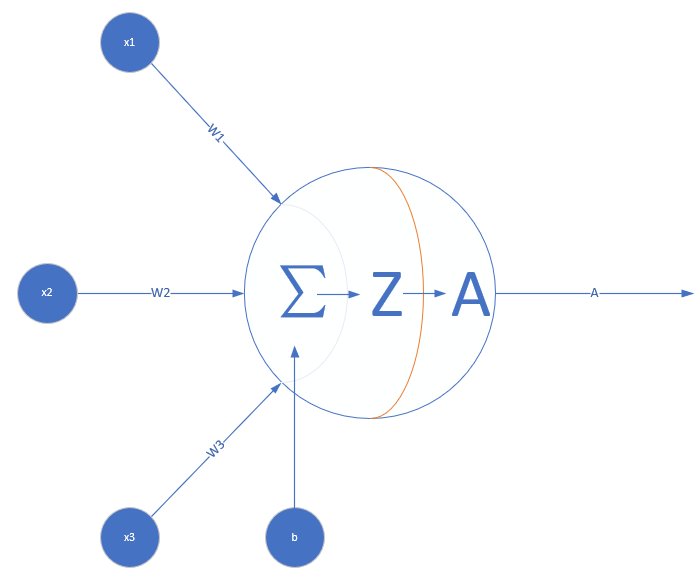• 从模仿人类大脑的角度来说：

• 说得形象一点，

if 严重等级 > 2: 老张打电话给隔壁老王寻求帮助 else: 自己解决

if 严重等级 > 6: 寻求物业公司帮助 else: 老王拿着管钳去老张家帮忙

• 从数学的角度来说
形如$z=\sum\limits_{i}w_ix_i+b_i$的传递是一种线性的传递过程。如果没有非线性函数添加非线性，直接把很多层叠加在一起会怎么样呢？
以两层为例：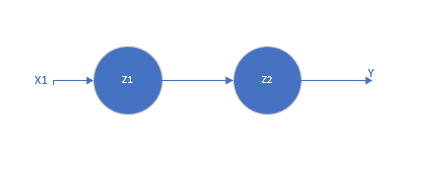$z_1 = w_1x_1 + b_1 \tag{1}$
$z_2 = w_2z_1 + b_2 = w_2(w_1x_1 + b_1) + b_2 = (w_2 w_1) x_1 + (w_2b_1 + b_2)=w_{3}x_1+b_{3} \tag{2}$

$z_1, z_2$即为这两个神经元结点的输出。可以看到，叠加后的$z_2$的输出也是一个线性函数。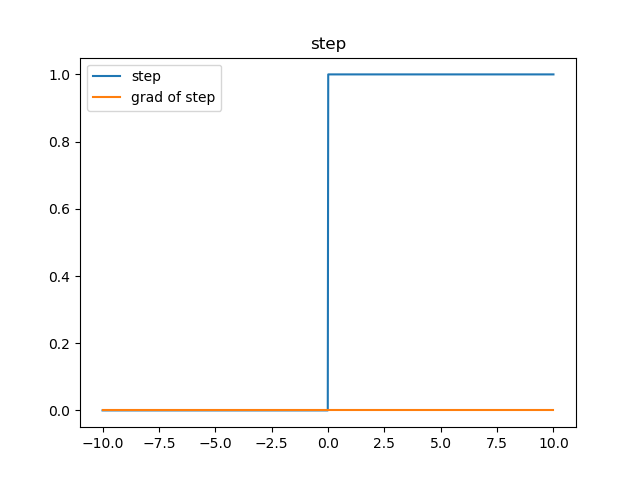sigmoid函数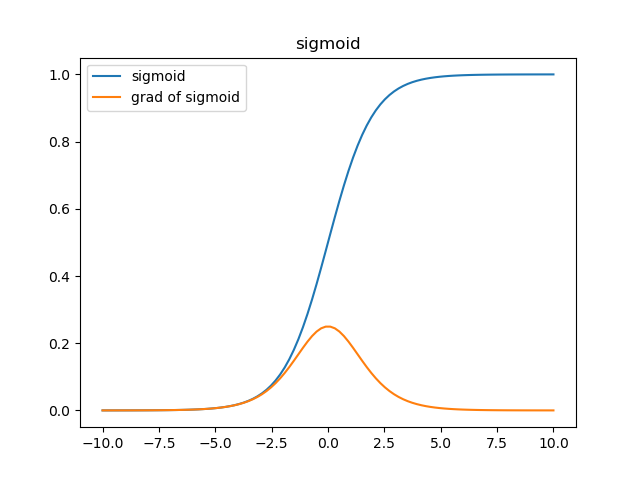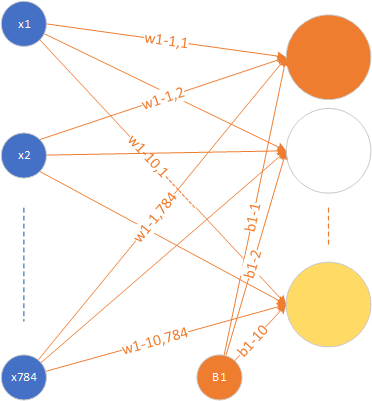$\frac{1}{1 + e^{-x}} = 0.9$
$e^{-x} = \frac{1}{9}$
$x = ln{9}$

$grad = f(x)\times(1 - f(x)) = 0.9 \times 0.1= 0.09$

$x_{new} = x - \eta \times grad = ln{9} - 0.2 \times 0.09 = ln(9) - 0.018$

$\frac{1}{1 + e^{-x_{new}}} = 0.898368$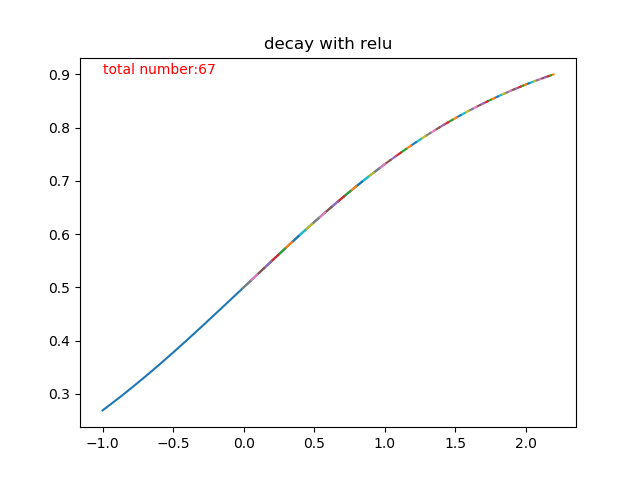class Csigmoid(object):
def __init__(self, inputSize):
self.shape = inputSize

def forward(self, image):
# 记录前向传播结果
self.mask = 1 / (1 + np.exp(-1 * image))

# 生成反向传播对应位置的梯度

tanh函数

$f(z) = \frac{e^{z} - e^{-z}}{e^{z} + e^{-z}}$
$f(z) = 2*sigmoid(2*z) - 1$

$f^{'}(z) = (1 + f(z)) * (1 - f(z))$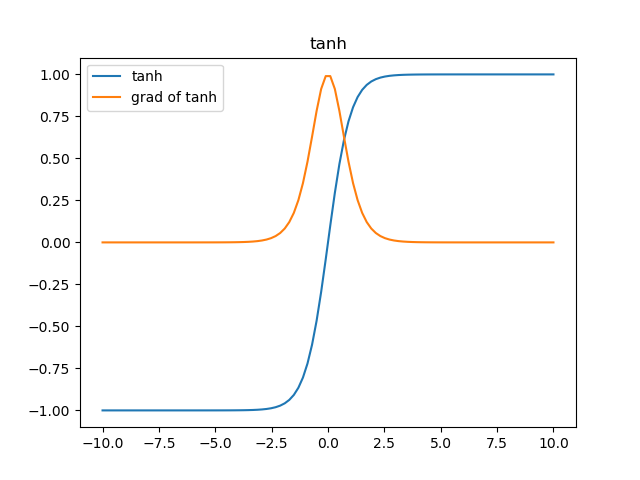class Ctanh(object):
def __init__(self, inputSize):
self.shape = inputSize

def forward(self, image):
# 记录前向传播结果
self.mask = 2 / (1 + np.exp(-2 * image)) - 1

# 生成反向传播对应位置的梯度

relu函数

$f(z) = \begin{cases} z & z \geq 0 \\ 0 & z < 0 \end{cases}$

$f(z) = \begin{cases} 1 & z \geq 0 \\ 0 & z < 0 \end{cases}$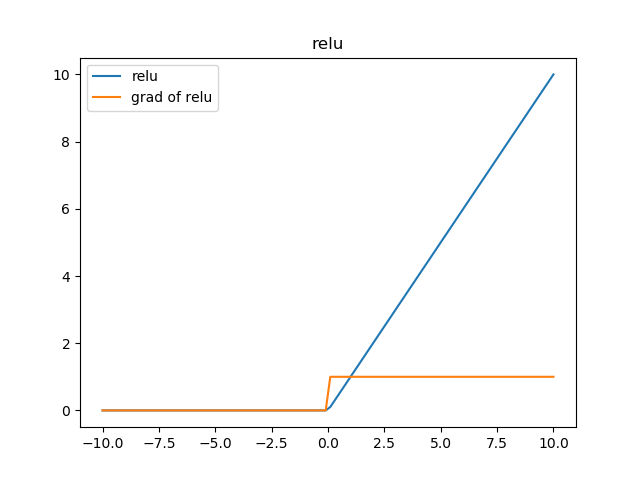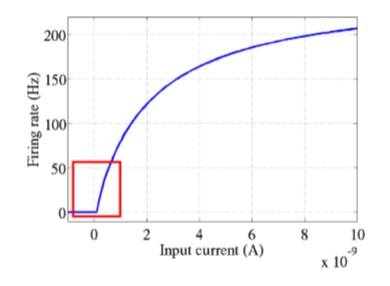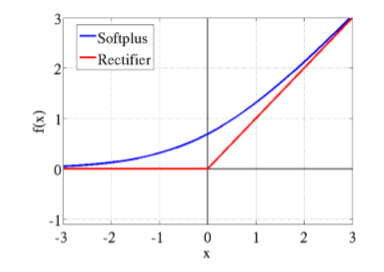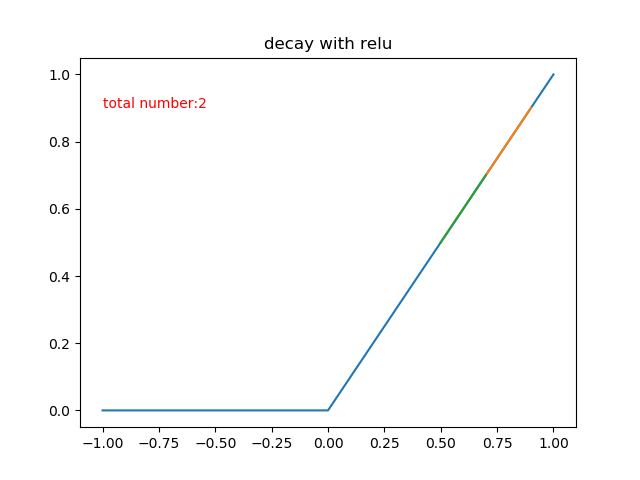class Crelu(object):
def __init__(self, inputSize):
self.shape = inputSize

def forward(self, image):
# 用于记录传递的结果
self.mask[image > 0] = 1
# 将小于0的项截止到0
return np.maximum(image, 0)

# 将上一层传递的误差函数和该层各位置的导数相乘

leaky relu函数

$f(z) = \begin{cases} z & z \geq 0 \\ \alpha * z & z < 0 \end{cases}$

$f(z) = \begin{cases} z & 1 \geq 0 \\ \alpha & z < 0 \end{cases}$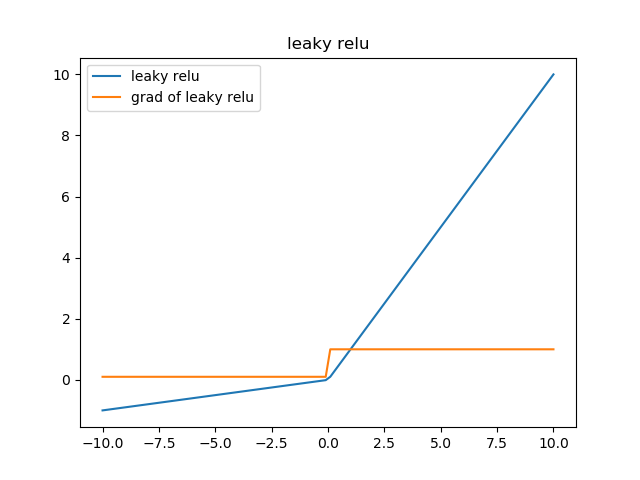class CleakyRelu(object):
def __init__(self, inputSize, alpha):
self.shape = inputSize
self.alpha = alpha

def forward(self, image):
# 用于记录传递的结果,按照传递公式生成对应的值
self.mask[image > 0] = 1
self.mask[image <= 0] = self.alpha
# 将该值对应到输入中

# 将上一层传递的误差函数和该层各位置的导数相乘

softmax 函数

softmax函数，是大名鼎鼎的在计算多分类问题时常使用的一个函数，他长成这个样子:

$\phi(z_j) = \frac{e^{z_j}}{\sum\limits_ie^{z_i}}$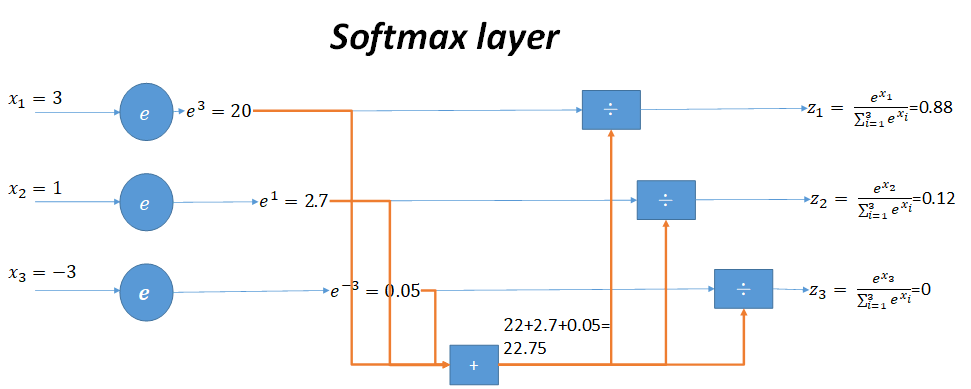$\phi(z_1) = \frac{e^{z_1}}{e^{z_1} + e^{z_2}} = \frac{1}{1 + e^{z_2 - z_1}} = \frac{1}{1 + e^{z_2} e^{- z_1}}$

$\phi(z_j) = \frac{e^{z_j}}{\sum\limits_ie^{z_i}} = \frac{e^{z_j}}{k + e^{z_j}}$

$\therefore \frac{\partial\phi(z_j)}{\partial z_j} = \frac{e^{z_j}(k + e^{z_j}) - e^{z_j} * e^{z_j}}{{(k + e^{z_j})}^2} = \frac{e^{z_j}}{k + e^{z_j}}\frac{k}{k + e^{z_j}} = softmax(z_j)(1 - softmax(z_j))$

[https://www.cnblogs.com/alexanderkun/p/8098781.html](https://www.cnblogs.com/alexanderkun/p/8098781.html)